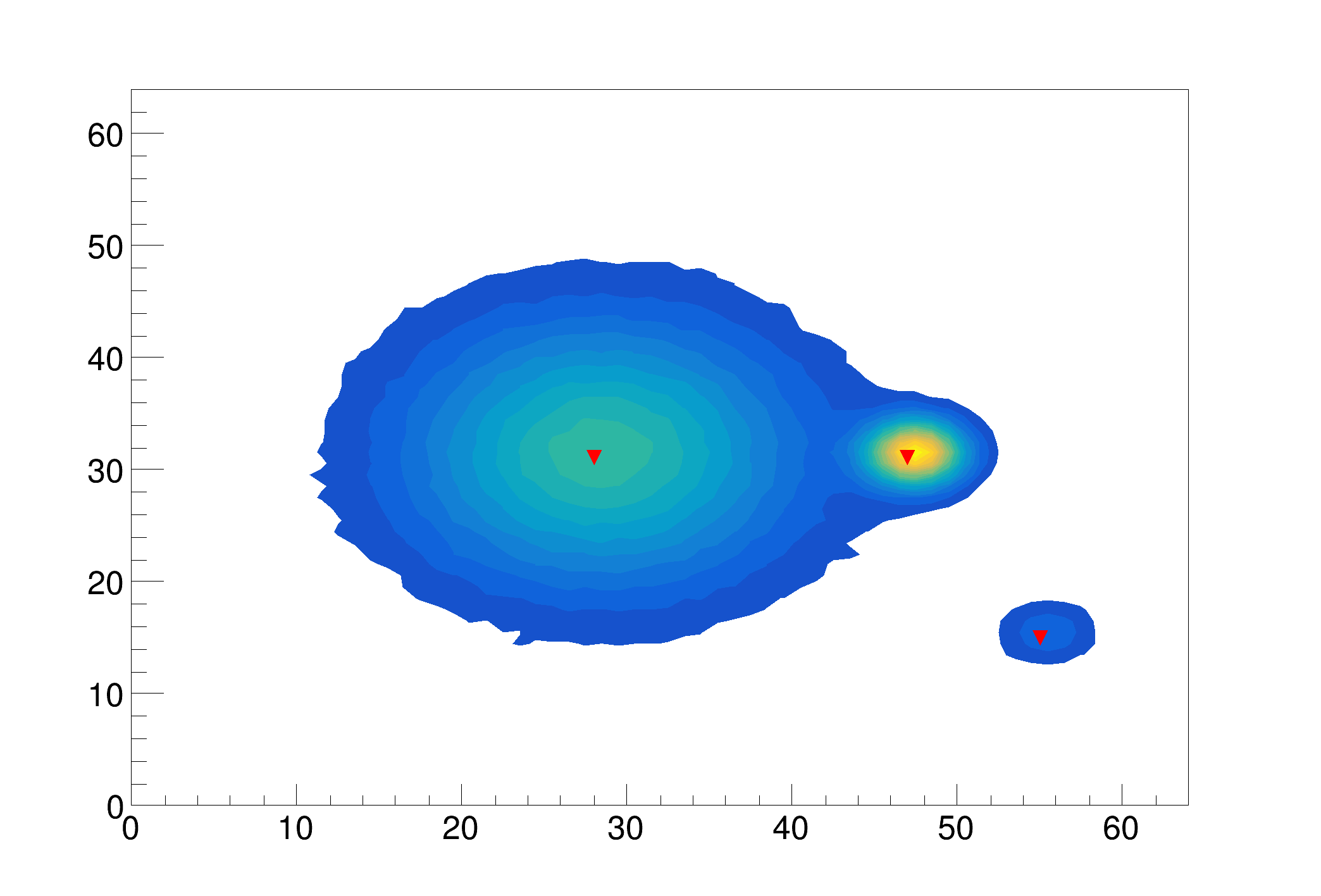ROOT   Reference GuideSrc4.C File Reference

## Detailed DescriptionExample to illustrate high resolution peak searching function (class TSpectrum2).Found 3 candidate peaks
posx= 47, posy= 31, value=10314
posx= 28, posy= 31, value=4994
posx= 55, posy= 15, value=1500
#include <TSpectrum2.h>
void Src4() {
Int_t i, j, nfound;
const Int_t nbinsx = 64;
const Int_t nbinsy = 64;
Double_t xmax = (Double_t)nbinsx;
Double_t ymax = (Double_t)nbinsy;
Double_t** source = new Double_t*[nbinsx];
for (i=0;i<nbinsx;i++)
source[i]=new Double_t[nbinsy];
Double_t** dest = new Double_t*[nbinsx];
for (i=0;i<nbinsx;i++)
dest[i]=new Double_t[nbinsy];
TString dir = gROOT->GetTutorialDir();
TString file = dir+"/spectrum/TSpectrum2.root";
TFile *f = new TFile(file.Data());
auto search = (TH2F*) f->Get("search2");
auto s = new TSpectrum2();
for (i = 0; i < nbinsx; i++){
for (j = 0; j < nbinsy; j++){
source[i][j] = search->GetBinContent(i + 1,j + 1);
}
}
nfound = s->SearchHighRes(source, dest, nbinsx, nbinsy, 3, 5, kFALSE, 10, kTRUE, 3);
printf("Found %d candidate peaks\n",nfound);
Double_t *PositionX = s->GetPositionX();
Double_t *PositionY = s->GetPositionY();
search->Draw("CONT");
auto m = new TMarker();
m->SetMarkerStyle(23);
m->SetMarkerColor(kRed);
for (i=0;i<nfound;i++) {
printf("posx= %d, posy= %d, value=%d\n",(Int_t)(PositionX[i]+0.5), (Int_t)(PositionY[i]+0.5),
(Int_t)source[(Int_t)(PositionX[i]+0.5)][(Int_t)(PositionY[i]+0.5)]);
m->DrawMarker(PositionX[i],PositionY[i]);
}
}
#define f(i)
Definition: RSha256.hxx:104
int Int_t
Definition: RtypesCore.h:45
const Bool_t kFALSE
Definition: RtypesCore.h:92
double Double_t
Definition: RtypesCore.h:59
const Bool_t kTRUE
Definition: RtypesCore.h:91
@ kRed
Definition: Rtypes.h:66
float xmin
Definition: THbookFile.cxx:95
float ymin
Definition: THbookFile.cxx:95
float xmax
Definition: THbookFile.cxx:95
float ymax
Definition: THbookFile.cxx:95
#define gROOT
Definition: TROOT.h:406
R__EXTERN TStyle * gStyle
Definition: TStyle.h:412
A ROOT file is a suite of consecutive data records (TKey instances) with a well defined format.
Definition: TFile.h:54
2-D histogram with a float per channel (see TH1 documentation)}
Definition: TH2.h:251
Manages Markers.
Definition: TMarker.h:22
Definition: TSpectrum2.h:18
Basic string class.
Definition: TString.h:136
void SetOptStat(Int_t stat=1)
The type of information printed in the histogram statistics box can be selected via the parameter mod...
Definition: TStyle.cxx:1589
static constexpr double s
Definition: file.py:1
auto * m
Definition: textangle.C:8
#define dest(otri, vertexptr)
Definition: triangle.c:1040

Definition in file Src4.C.